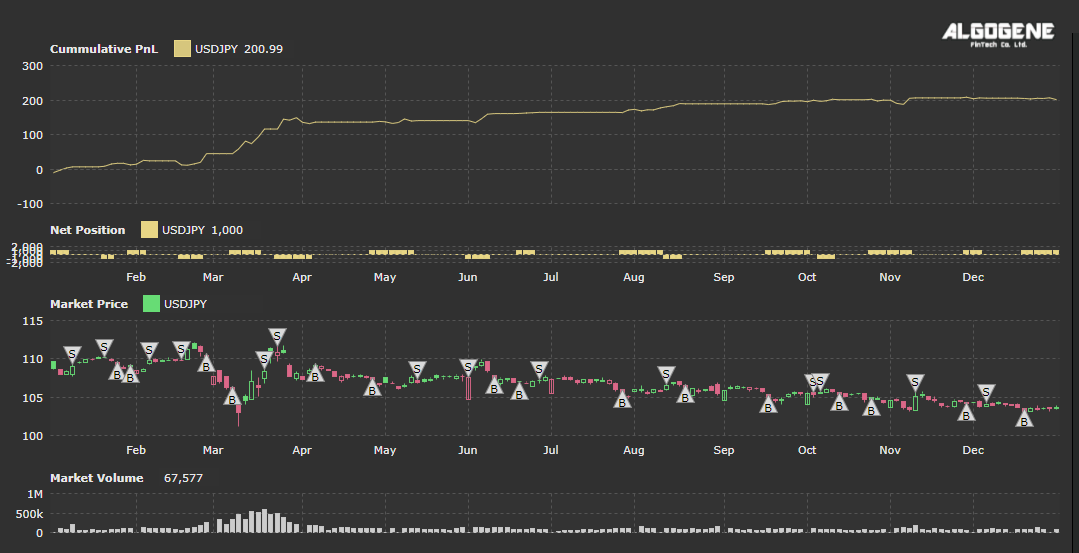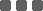## A simple RSI strategy

Following the discussion from the previous post [Technical Indicator - Relative Strength Index (RSI)], we will implement a simple trading strategy using the RSI indicator.

Suppose we apply RSI(14) for the daily closing price of USDJPY over the year of 2020.

• Based on a sliding window approach to collect the previous 14 closing price
• Calculate the latest RSI value
• Order open conditions:
• if we have NO outstanding position,
• if RSI value < 30, we open a buy order
• if RSI value > 70, we open a sell order
• Order close conditions:
• if we have outstanding position,
• if we previously submit a buy order and RSI value reverses back to or above 50, we close the buy order
• if we previously submit a sell order and RSI value reverses back to or below 50, we close the sell order
• Repeat the process until the backtest period end

Now, let's write down our trading algorithm step-by-step.

### Step 1. Calculate RSI value

First of all, we define 'rsi_period' and 'instrument' at initialization.

 ``` 1 2 3 4 5 6 7 8 9 10``` ```from AlgoAPI import AlgoAPIUtil, AlgoAPI_Backtest from datetime import datetime, timedelta from talib import RSI import numpy as np class AlgoEvent: def __init__(self): self.timer = datetime(1970,1,1) self.rsi_period = 14 self.instrument = "USDJPY" ```

We use the API function getHistoricalBar to collect historical observations. Then, we apply a python library 'talib.RSI' to calculate the latest RSI value.

 ``` 1 2 3 4 5 6 7 8 9 10 11 12 13 14 15``` ``` def on_marketdatafeed(self, md, ab): # execute stratey every 24 hours if md.timestamp >= self.timer+timedelta(hours=24): # get last 14 closing price res = self.evt.getHistoricalBar({"instrument":self.instrument}, self.rsi_period+1, "D") arr = [res[t]['c'] for t in res] # calculate the current RSI value RSI_cur = RSI(np.array(arr), self.rsi_period)[-1] # print out RSI value to console self.evt.consoleLog("RSI_cur = ", RSI_cur) # update timer self.timer = md.timestamp ```

### Step 2. Order Entry Conditions

We further define 'rsi_overbought', 'rsi_oversold' and 'position' at initialization.

 ``` 1 2 3 4 5 6 7 8 9 10 11 12 13 14``` ```from AlgoAPI import AlgoAPIUtil, AlgoAPI_Backtest from datetime import datetime, timedelta from talib import RSI import numpy as np class AlgoEvent: def __init__(self): self.timer = datetime(1970,1,1) self.rsi_period = 14 self.rsi_overbought = 70 self.rsi_oversold = 30 self.position = 0 self.instrument = "USDJPY" ```

We also create a function 'open_order' to handle order submissions.

 ```1 2 3 4 5 6 7 8``` ``` def open_order(self, buysell): order = AlgoAPIUtil.OrderObject() order.instrument = self.instrument order.openclose = 'open' order.buysell = buysell #1=buy, -1=sell order.ordertype = 0 #0=market, 1=limit order.volume = 0.01 self.evt.sendOrder(order) ```

We also update the system's function 'on_marketdatafeed' for order open logic.

 ``` 1 2 3 4 5 6 7 8 9 10 11 12 13 14 15 16 17 18 19 20 21 22 23 24 25 26``` ``` def on_marketdatafeed(self, md, ab): # execute stratey every 24 hours if md.timestamp >= self.timer+timedelta(hours=24): # get last 14 closing price res = self.evt.getHistoricalBar({"instrument":self.instrument}, self.rsi_period+1, "D") arr = [res[t]['c'] for t in res] # calculate the current RSI value RSI_cur = RSI(np.array(arr), self.rsi_period)[-1] # print out RSI value to console self.evt.consoleLog("RSI_cur = ", RSI_cur) # open an order if we have no outstanding position if self.position==0: # open a sell order if it is overbought if RSI_cur>self.rsi_overbought: self.open_order(-1) # open a buy order if it is oversold elif RSI_cur

### Step 3. Order Close Condition

We firstly edit the system's 'on_orderfeed' function. When there is order status update from the system, we record the system tradeID and update our outstanding position.

 ```1 2 3 4 5 6 7 8``` ``` def on_orderfeed(self, of): # when system confirm an order, update last_tradeID and position if of.status=="success": self.position += of.fill_volume*of.buysell if self.position==0: self.last_tradeID = "" else: self.last_tradeID = of.tradeID ```

Secondly, we create a 'close_order' function.

 ```1 2 3 4 5``` ``` def close_order(self): order = AlgoAPIUtil.OrderObject() order.openclose = 'close' order.tradeID = self.last_tradeID self.evt.sendOrder(order) ```

Finally, we update 'on_marketdatafeed' function for order closing logic.

 ``` 1 2 3 4 5 6 7 8 9 10 11 12 13 14 15 16 17 18 19 20 21 22 23 24 25 26 27 28 29 30 31 32 33 34 35 36 37``` ``` def on_marketdatafeed(self, md, ab): # execute stratey every 24 hours if md.timestamp >= self.timer+timedelta(hours=24): # get last 14 closing price res = self.evt.getHistoricalBar({"instrument":self.instrument}, self.rsi_period+1, "D") arr = [res[t]['c'] for t in res] # calculate the current RSI value RSI_cur = RSI(np.array(arr), self.rsi_period)[-1] # print out RSI value to console self.evt.consoleLog("RSI_cur = ", RSI_cur) # open an order if we have no outstanding position if self.position==0: # open a sell order if it is overbought if RSI_cur>self.rsi_overbought: self.open_order(-1) # open a buy order if it is oversold elif RSI_cur0 and RSI_cur>50: self.close_order() # close a position if we have previously open a sell order and RSI now reverse below 50 elif self.position<0 and RSI_cur<50: self.close_order() # update timer self.timer = md.timestamp ```

### Full Source Code

Combining all above, the full script is presented below.

 ``` 1 2 3 4 5 6 7 8 9 10 11 12 13 14 15 16 17 18 19 20 21 22 23 24 25 26 27 28 29 30 31 32 33 34 35 36 37 38 39 40 41 42 43 44 45 46 47 48 49 50 51 52 53 54 55 56 57 58 59 60 61 62 63 64 65 66 67 68 69 70 71 72 73 74 75 76 77 78 79 80 81 82 83 84 85 86 87 88 89 90 91 92 93 94 95 96 97 98 99 100 101 102``` ```from AlgoAPI import AlgoAPIUtil, AlgoAPI_Backtest from datetime import datetime, timedelta from talib import RSI import numpy as np class AlgoEvent: def __init__(self): self.timer = datetime(1970,1,1) self.rsi_period = 14 self.rsi_overbought = 70 self.rsi_oversold = 30 self.position = 0 self.last_tradeID = "" self.instrument = "USDJPY" def start(self, mEvt): self.evt = AlgoAPI_Backtest.AlgoEvtHandler(self, mEvt) self.evt.start() def on_marketdatafeed(self, md, ab): # execute stratey every 24 hours if md.timestamp >= self.timer+timedelta(hours=24): # get last 14 closing price res = self.evt.getHistoricalBar({"instrument":self.instrument}, self.rsi_period+1, "D") arr = [res[t]['c'] for t in res] # calculate the current RSI value RSI_cur = RSI(np.array(arr), self.rsi_period)[-1] # print out RSI value to console self.evt.consoleLog("RSI_cur = ", RSI_cur) # open an order if we have no outstanding position if self.position==0: # open a sell order if it is overbought if RSI_cur>self.rsi_overbought: self.open_order(-1) # open a buy order if it is oversold elif RSI_cur0 and RSI_cur>50: self.close_order() # close a position if we have previously open a sell order and RSI now reverse below 50 elif self.position<0 and RSI_cur<50: self.close_order() # update timer self.timer = md.timestamp def open_order(self, buysell): order = AlgoAPIUtil.OrderObject() order.instrument = self.instrument order.openclose = 'open' order.buysell = buysell #1=buy, -1=sell order.ordertype = 0 #0=market, 1=limit order.volume = 0.01 self.evt.sendOrder(order) def close_order(self): order = AlgoAPIUtil.OrderObject() order.openclose = 'close' order.tradeID = self.last_tradeID self.evt.sendOrder(order) def on_bulkdatafeed(self, isSync, bd, ab): pass def on_newsdatafeed(self, nd): pass def on_weatherdatafeed(self, wd): pass def on_econsdatafeed(self, ed): pass def on_orderfeed(self, of): # when system confirm an order, update last_tradeID and position if of.status=="success": self.position += of.fill_volume*of.buysell if self.position==0: self.last_tradeID = "" else: self.last_tradeID = of.tradeID def on_dailyPLfeed(self, pl): pass def on_openPositionfeed(self, op, oo, uo): pass ```

### Results

Now, we are prepared to backtest this strategy.

Backtest Settings:

• Instrument: USDJPY
• Period: 2020.01 - 2020.12
• Initial Capital: US\$1,000
• Data Interval: 1-day bar
• Allow Shortsell: True

Backtest Result### Final Thoughts

Although the backtest result above looks promising, some questions are raised below to brainstorm how to further make this strategy practical.

• From the logics above, we assume short selling is possible. What if it is not feasible in stock market?
• We are using a 14-day RSI.
• Any better choice than 14?
• Is it applicable to other timeframes, eg. 1-min, 1-hour?
• Will multiple RSIs produce a more reliable result?
• The RSI value of 30/70 are used as reference points for order entry.
• Any other better combinations?
• Can this entry points be dynamically changing?
• Can the 30/70 rule generally applicable to other financial instruments and asset classes?
• In our order exit logic, we close a trade when RSI reverses back to 50.
• Any better choice than 50?
• We should also think about stop loss conditions.
• Suppose we enter a buy order when RSI is low. If that instrument's price keep droping, its calculated RSI will maintain at a low level and won't reverse back to 50. Thus, according to current trading logics, we will keep losing money and never cut loss.
• Will it produce a more accurate signal when combining RSI with other indicators?

wow, it has a beautiful P/L curve :)
RSI is a very useful indicator!

### Categories

Hot Topic

Introduce the ALGOGENE Affiliate Program

23 0 0 2023-03-24

The pros and cons of ChatGPT in FinTech

57 0 1 2023-03-13

Implement Grid Trading Strategy in Python

132 0 1 2023-03-07

259 2 5 2023-03-01

Guideline for Strategy Parameter Optimizer

239 1 2 2023-02-12

106 1 0 2023-02-08

264 3 2 2023-02-03

Request for parameter optimization function

146 3 1 2023-01-21